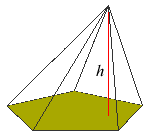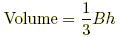index: click on a letter A B C D E F G H I J K L M N O P Q R S T U V W X Y Z A to Z index index: subject areas numbers & symbols sets, logic, proofs geometry algebra trigonometry advanced algebra & pre-calculus calculus advanced topics probability & statistics real world applications multimedia entrieswww.mathwords.com about mathwords website feedback

Regular Pyramid

A pyramid with a base that is a regular polygon. The apex is not necessarily directly above the center of the base.

Note: For some mathematicians regular pyramid means the same as right regular pyramid.Solid view: regular pyramid with a regular pentagon as baseFrame view: regular pyramid with a regular pentagon as baseh = height of the pyramid B = area of the base